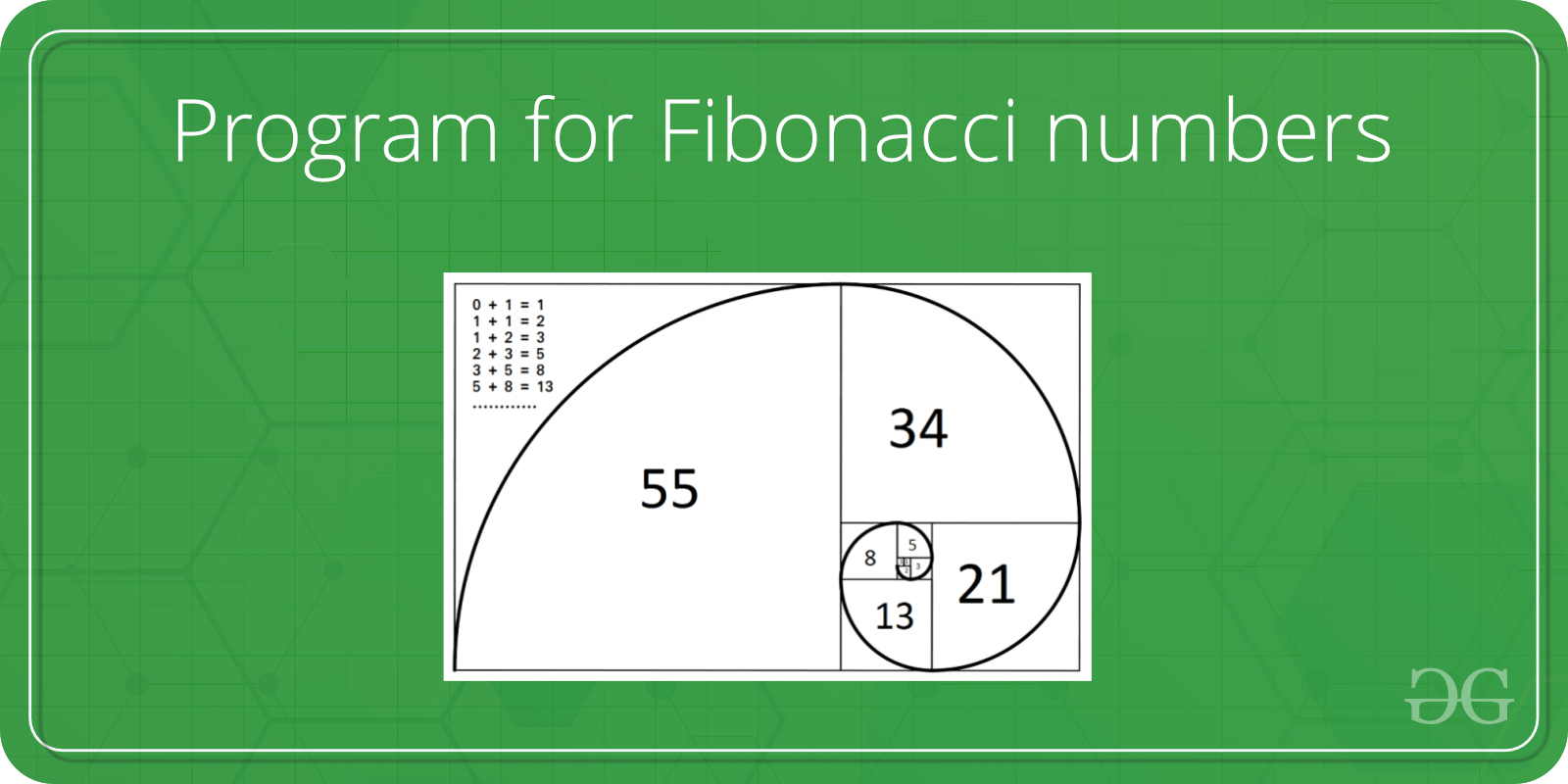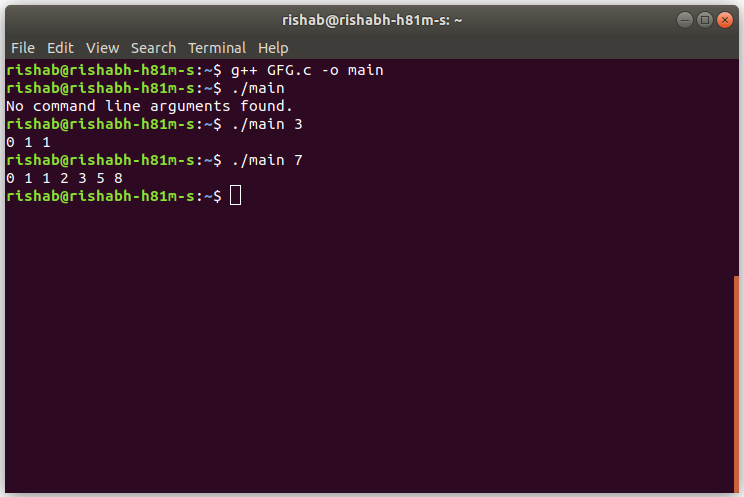Open in App
Not now

# TCS Coding Practice Question | Fibonacci Series

• Difficulty Level : Medium
• Last Updated : 03 Nov, 2022

Given a number ‘n’, the task is to print the Fibonacci series using Command Line Arguments. The Fibonacci numbers are the numbers in the following integer sequence.

0, 1, 1, 2, 3, 5, 8, 13, 21, 34, 55, 89, 144, ……..

In mathematical terms, the sequence Fn of Fibonacci numbers is defined by the recurrence relation

`    Fn = Fn-1 + Fn-2`

with seed values

`   F0 = 0 and F1 = 1.`Examples:

```Input: n = 3
Output: 0, 1, 1

Input: 7
Output: 0, 1, 1, 2, 3, 5, 8```Approach:

• Since the number is entered as Command line Argument, there is no need for a dedicated input line
• Extract the input number from the command line argument
• This extracted number will be in String type.
• Convert this number into integer type and store it in a variable, say num
• Now the function fib having num value will work.
• In this function now take three variables a, b and c and now store two of them value as a=0, b=1;
• Now if the num ==1, then there will be no fibonacci number return a.
• If num >1 then we will swap the all variable values like as
• c=a+b
• a = b
• b = c
• Now we will just print the all values a, b and c.

Program:

## C

 `// C program to print the Fibonacci Series ` `// using command line arguments ` ` `  `#include ` `#include /* atoi */ ` ` `  `// Function to print the Fibonacci Series ` `void` `fib(``int` `n) ` `{ ` ` `  `    ``int` `a = 0, b = 1, c, i; ` ` `  `    ``if` `(n <= 1) ` `        ``printf``(``"%d "``, a); ` `    ``else` `{ ` ` `  `        ``printf``(``"%d %d "``, a, b); ` ` `  `        ``for` `(i = 3; i <= n; i++) { ` `            ``c = a + b; ` `            ``a = b; ` `            ``b = c; ` ` `  `            ``printf``(``"%d "``, c); ` `        ``} ` ` `  `        ``printf``(``"\n"``); ` `    ``} ` `} ` ` `  `// Driver code ` `int` `main(``int` `argc, ``char``* argv[]) ` `{ ` ` `  `    ``int` `num, res = 0; ` ` `  `    ``// Check if the length of args array is 1 ` `    ``if` `(argc == 1) ` `        ``printf``(``"No command line arguments found.\n"``); ` ` `  `    ``else` `{ ` ` `  `        ``// Get the command line argument and ` `        ``// Convert it from string type to integer type ` `        ``// using function "atoi( argument)" ` `        ``num = ``atoi``(argv); ` ` `  `        ``// Print the Fibonacci series ` `        ``fib(num); ` `    ``} ` `    ``return` `0; ` `} `

## Java

 `// Java program to print the Fibonacci Series ` `// using command line arguments ` ` `  `class` `GFG { ` ` `  `    ``// Function to print the Fibonacci Series ` `    ``static` `void` `fib(``int` `n) ` `    ``{ ` ` `  `        ``int` `a = ``0``, b = ``1``, c, i; ` ` `  `        ``if` `(n <= ``1``) ` `            ``System.out.print(a + ``" "``); ` `        ``else` `{ ` ` `  `            ``System.out.print(a + ``" "` `+ b + ``" "``); ` ` `  `            ``for` `(i = ``3``; i <= n; i++) { ` `                ``c = a + b; ` `                ``a = b; ` `                ``b = c; ` ` `  `                ``System.out.print(c + ``" "``); ` `            ``} ` ` `  `            ``System.out.println(); ` `        ``} ` `    ``} ` ` `  `    ``// Driver code ` `    ``public` `static` `void` `main(String[] args) ` `    ``{ ` ` `  `        ``// Check if length of args array is ` `        ``// greater than 0 ` `        ``if` `(args.length > ``0``) { ` ` `  `            ``// Get the command line argument and ` `            ``// Convert it from string type to integer type ` `            ``int` `num = Integer.parseInt(args[``0``]); ` ` `  `            ``// Print the Fibonacci series ` `            ``fib(num); ` `        ``} ` `        ``else` `            ``System.out.println(``"No command line "` `                               ``+ ``"arguments found."``); ` `    ``} ` `} `

Output:

• In C:• In Java:Time Complexity: O(N)
Auxiliary Space: O(1)

My Personal Notes arrow_drop_up
Related Articles